# Transforming predicted variables in regression

The other day on LinkedIn I made a point about how I think scikits `TransformedTargetRegressor` is very likely to mislead folks. In fact, the example use case in the docs for this function is a common mistake, fitting a model for `log(y)`, then getting predictions `phat`, and then simply exponentiating those predictions `exp(phat)`.

On LinkedIn I gave an example of how this is problematic for random forests, but here is a similar example for linear regression. For simplicity pretend we only have 3 potential residuals (all equally likely), either a residual of -1, 0, or 1.

Now pretend our logged prediction is 5, so if we simply do `exp(5)` we get about `148`. Now what are our predictions is we consider those 3 potential residuals?

``````Resid  Pred-Resid Modified_Pred LinPred
-1     5 - -1        exp(6)     403
0     5 -  0        exp(5)     148
1     5 -  1        exp(4)      55``````

So if we take the mean of our `LinPred` column, we then get a prediction of about `202`. The prediction using this approach is much higher than the naive approach of simply exponentiating 5. The difference is that the exp(5) estimate is the median, and the above estimate taking into account residuals is the mean estimate.

While there are some cases you may want the median estimate, in that case it probably makes more sense to use a quantile estimator of the median from the get go, as opposed to doing the linear regression on `log(y)`. I think for many (probably most) use cases in which you are predicting dollar values, this underestimate can be very problematic. If you are using these estimates for revenue, you will be way under for example. If you are using these estimates for expenses, holy moly you will probably get fired.

This problem will happen for any non-linear transformation. So while some transformations are ok, in scikit for example minmax or standardnormal scalars are ok, things like logs, square roots, or box-cox transformations are not. (To know if it is a linear transformation, if you do a scatterplot of original vs transformed, if it is a straight line it is ok, if it is a curved line it is not!)

I had a friend go back and forth with me for a bit after I posted this. I want to be clear this is not me saying the model of `log(y)` is the wrong model, it is just to get the estimates for the mean predictions, you need to take a few steps. In particular, one approach to get the mean estimates is to use Duan’s Smearing estimator. I will show how to do that in python below using simulated data.

# Example Duan’s Smearing in python

So first, we import the libraries we will be using. And since this is simulated data, will be setting the seed as well.

``````######################################################
import pandas as pd
import numpy as np
np.random.seed(10)

from sklearn.linear_model import LinearRegression
from sklearn.compose import TransformedTargetRegressor
######################################################``````

Next I will create a simple linear model on the log scale. So the regression of the logged values is the correct one.

``````######################################################
# Make a fake dataset, say these are housing prices
n = (10000,1)
error = np.random.normal(0,1,n)
x1 = np.random.normal(10,3,n)
x2 = np.random.normal(5,1,n)
log_y = 10 + 0.2*x1 + 0.6*x2 + error
y = np.exp(log_y)

dat = pd.DataFrame(np.concatenate([y,x1,x2,log_y,error], axis=1),
columns=['y','x1','x2','log_y','error'])
x_vars = ['x1','x2']

# Lets look at a histogram of y vs log y
dat['y'].hist(bins=100)
dat['log_y'].hist(bins=100)
######################################################``````

Here is the histogram of the original values: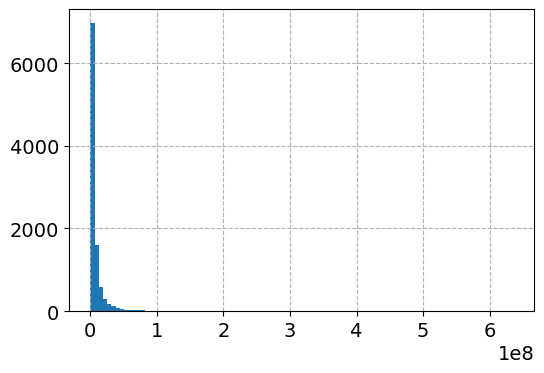And here is the histogram of the logged values: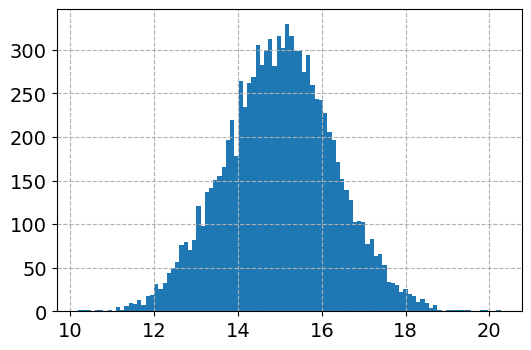So although the regression is the conditional relationship, if you see histograms like this I would also by default use a regression to predict `log(y)`.

Now here I do the same thing as in the original function docs, I fit a linear regression using the log as the function and exponential as the inverse function.

``````######################################################
# Now lets see what happens with the usual approach
tt = TransformedTargetRegressor(regressor=LinearRegression(),
func=np.log, inverse_func=np.exp)
tt.fit(dat[x_vars], dat['y'])
print( (tt.regressor_.intercept_, tt.regressor_.coef_) ) #Estimates the correct values

dat['WrongTrans'] = tt.predict(dat[x_vars])

dat[['y','WrongTrans']].describe()
######################################################``````

So here we estimate the correct simulated values for the regression equation: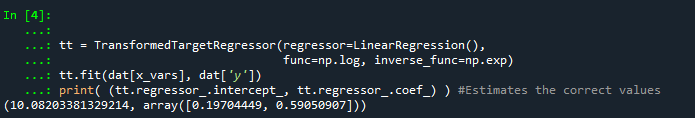But as we will see in a second, the exponentiated predictions are not so well behaved. To illustrate how the `WrongTrans` variable behaves, I show its distribution compared to the original `y` value. You can see that on average it is a much smaller estimate. Our sample values have a mean of 7.5 million, and the naive estimate here only has a mean of 4.6 million.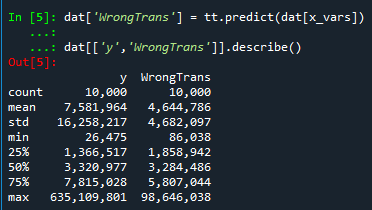Now here is a way to get an estimate of the mean value. In a nutshell, what you do is take the observed residuals, pretty much like that little table I did in the intro of this blog post, generate predictions given those residuals, and then back transform them and take the mean.

Although this example is using logged regression, I’ve made it pretty general. So if you used any box cox transformation instead of the logged (e.g. sklearns power_transform, it will work.

``````######################################################
# Duan's smearing, non-parametric approach via residuals

# Can make this general for any function inside of
# TransformedTargetRegressor
f = tt.get_params()['func']              #function
inv_f = tt.get_params()['inverse_func']  #and inverse function

# Non-parametric approach, approximate via residuals
log_pred = f(dat['WrongTrans'])
resids = f(dat['y']) - log_pred
resids = resids.values.reshape(1,n)
dp = inv_f(log_pred.values.reshape(n,1) + resids)
dat['DuanPreds'] = dp.mean(axis=1)

dat[['y','WrongTrans','DuanPreds']].describe()
######################################################``````

So you can see that the Duan Smeared predictions are looking better, at least the mean of the predictions is much closer to the original.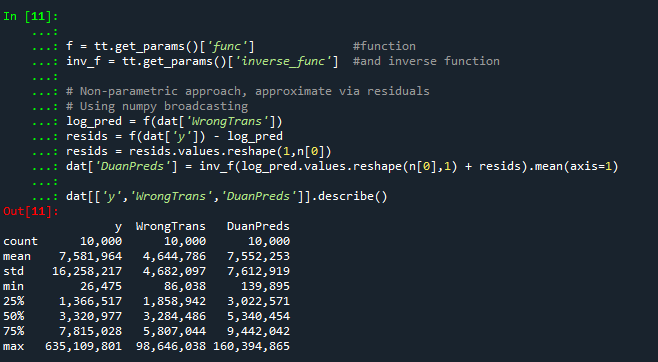I’ve intentionally done this example without using train/test, as we know the true answers. But in that case, you will want to use the residuals from the training dataset to apply this transformation to the test dataset.

So the residuals and the Duan smearing estimator do not need to be the same dimension. So for example if you have a big data application, you may want to do something like `resids = resids.sample(1000)` above.

Also another nice perk of this is you can use `dp` above to give you prediction intervals, so `np.quantile(dp,[0.025,0.975], axis=1).T` would give you a 95% prediction interval of the mean on the linear scale as well.

# Extra, Parametric Estimation

Another approach, which may make sense given the application, is instead of using the observed residuals to give a non-parametric estimate, you can estimate the distribution of the residuals, and then use that to make either an integral estimate of the Smeared estimate back on the original scale. Or in the case of the logged regression there is a closed form solution.

I show how to construct the integral estimator below, again trying to be more general. The integral approach will work for say any box-cox transformation.

``````######################################################
# Parametric approach, approximating residuals via normal

from scipy.stats import norm

# Look at the residuals again
resids = f(dat['y']) - f(tt.predict(dat[x_vars]))

# Check to make sure that the residuals are really close to normal
# Before doing this
resids.hist(bins=100)

# Fit to a normal distribution
loc, scale = norm.fit(resids)

# Define integral
def integrand(x,pred):
return norm.pdf(x, loc, scale)*inv_f(pred - x)

# Pred should be the logged prediction
# -50,50 should be changed if the residuals are scaled differently
def duan_param(pred):

# This takes awhile to apply to the whole data frame!
dat['log_pred'] = f(tt.predict(dat[x_vars]))
sub_dat['DuanParam'] = sub_dat['log_pred'].apply(duan_param)

# Can see that these are very similar to the non-parametric

And you can see that this normal based approximation works just fine here, since by construction the model residuals are pretty well behaved in my simulation.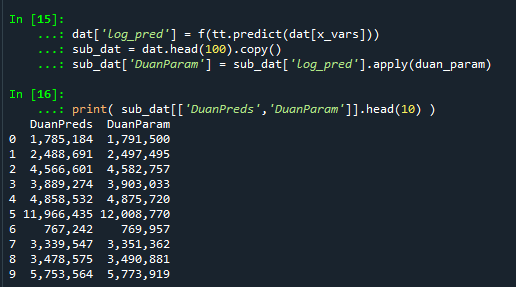It happens to be the case that there is a simpler estimate than the integral approach (which you can see in my notes takes awhile to estimate).

``````###########
# Easier way, but only applicable to log transform
# https://en.wikipedia.org/wiki/Smearing_retransformation
test_val = np.log(5000000)

# Integral approach
print( duan_param(test_val) )

# Approach for just log transformed
mult = np.exp(0.5*resids.var())
print( np.exp(test_val)*mult )
##########``````

So you can see the integral vs the closed form function are very close: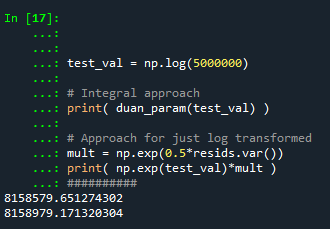The differences could be due to the the integral is simply an estimate (and you can see I did not do negative to positive infinity, but chopped it off, I do not know if there is a better function to estimate the integral or general approach here).

It wouldn’t surprise me if there are closed form solutions for box-cox transforms as well, but I am not familiar with them offhand. Again the integral approach (or the non-parametric approach) will work for whatever function you want. The function itself could be whatever crazy/discontinuous function you want. But this parametric Duan’s Smearing approach relies on the residuals being normally distributed. (I suppose you could use some other types of continuous distribution estimate if you have reason to, I have only seen normal distribution estimates though in practice.)

# Other Notes

While this focuses on regression, I do not think this will perform all that badly for other types of models (such as random forests or xgboost). But for forests it may make sense to simply pull out the individual tree estimates, back transform them, and get the mean of that backtransformed estimate. I have a different blog post that has a function showing how to scoop up the individual predictions from a random forest model.

It should also apply the same to any regression model with regularization. But if you want to do this, there are of course other alternative models you may consider that may be better suited towards your end goals of predictions on the linear/original scale.

For example, if you really want prediction intervals, it may make sense to not transform the data, and estimate a quantile regression model at the 5% and 95% quantiles. This would give you a 90% prediction interval.

Another approach is that it may make sense to use a different model, such as Poisson regression or negative binomial regression (or another generalized linear model in general). Even if your data are not integer counts, you can still use these models! (They just need to be 0 and above, no negative values.)

That Stata blog suggests to use Poisson and then robust standard errors, but that is a bad idea if you are really interested in predictions as well (see Gary Kings comment and linked paper). But you can just do negative binomial models in most cases then, and that is a better default than Poisson for many real world datasets.

# Regression with Simple Weights

I was reminded of this paper by Jung et al. on constructing simple rules via regression recently. So in the past few posts I have talked about how RTM (1,2) is aimed at making simple models. This is via variable selection and/or simplying the inputs to be binary yes/no. But in the end the final equation could be something like:

``log(Crime) = -0.56 + 0.6923*NearbyBars + 0.329*HighDensity311``

The paper linked above is about making the regression weights simple, so instead of a regression weight of 0.89728, you may just round the regression weight to 1. The Jung paper does a procedure where they use lasso regression and then round the weights. But there is a simpler approach IMO I will illustrate, just amend the lasso weights to push the coefficients to simple integers. (Also reminded by this example of using an iterative linear program to push weights to binary 0/1.)

So in lasso, you estimate your normal regression equation, but put a penalty on the weights that is typically something like `lambda*(sum(abs(reg_weights)) - 1)**2`. So if you have reg weights that add to more than 1, they are penalized by a particular amount (the lambda is a tuner to make the penalty higher/lower). And in the iterative algorithm to minimize your loss function plus this added penalty, it will converge to regression weights that meet the criteria of in total summing to around 1. Not exactly 1 but close.

You can however swap out that penalty term with whatever you want (or add to it additional penalties). I will show an example of using a penalty term to push regression coefficients towards integer values, creating simple regression weights.

# Why Simple Models?

Dan Simpson has a good blog post of the Jung paper and why simple models are sometimes preferable (and I also have a comment why simple models like this tend to work out well for CJ datasets). But here are few quick examples why you might want a simple model results.

Example 1: If you have people in the field who are tabulating data and making quick decisions, it may be they need to use pen/paper and make a quick decision. No time to input results into a computer and pop out a prediction. Imagine a nurse in the ER, or even your general practitioner. There may be quite a bit of utility in making a simple check list that says `if +4 on this scale, do a more intensive treatment`.

Example 2: You have a complicated, large database. It is easier to create a simple predictive model in SQL to serve up predictions (either because of latency or because of the complexity of the data pipeline). Instead of a complicated random forest, a linear regression with simple weights will be much easier to implement.

Example 3: Transparency. Complicated models are more difficult to understand and monitor. If you have a vested interest in presenting the model to outside parties, it may make sense to sacrifice some accuracy to make the model more interpretable. Also similar to lasso, I suspect these simple weights will reduce the variance of predictions.

The reason that these simple weights work well in practice for many social science examples you could interpret either in a good light or a bad one. For the half-empty interpretation, our models are not well identified – we can literally swap out various weights in our regression equation and get near similar predictions. So it is fools errand to try to find the regression equation that describes the underlying system. But you can flip that around as well, we don’t even need to find the perfect equation, we can identify quite a few good predictive equations. And why not pick a good equation that is easier to interpret?

# Pytorch Example

The example set of code here is very simple, so I will just put the python code entirely in this post. First I import my libraries I am using and change my directory.

``````############################################
import os
import torch
import statsmodels.api as sm
import statsmodels.formula.api as smf
import pandas as pd
import numpy as np

my_dir = r'D:\Dropbox\Dropbox\Documents\BLOG\regression_simpleweights\analysis'
os.chdir(my_dir)
############################################``````

Next I read in the data, which I have previously used as an example in prior blog posts on doctor visits for medicare patients. One thing to note here, is that I rescale the independent variables I am using to min/max. So the age variable instead of going from 65-90 like in the original data, now is scaled to be between 0/1. This is a problem intrinsic to lasso as well, in that you can change the scale of the input variables and it changes the weights. Here with the original data, the education variable has a tiny regression coefficient (0.2), but is highly stat significant. So without rescaling that variable, the model said to hell with your penalty and still converged to a solution of that regression weight is 0.2. If you divide the education variable by 5 though, the corresponding regression weight would change to around 1.

``````###########################################
#Data from Stata, https://www.stata-press.com/data/r16/gsem_mixture.dta
#see pg 501 https://www.stata.com/manuals/sem.pdf

y_dat = visit_dat[['drvisits']]
x_vars = ['private','medicaid','age','educ','actlim','chronic']
#rescaling variables to 0/1
x_dat = visit_dat[x_vars]
visit_dat[x_vars] = (x_dat - x_dat.min(axis=0)) / ( x_dat.max(axis=0)  - x_dat.min(axis=0) )
x_dat = visit_dat[x_vars] #intentional not a copy
###########################################``````

Now in the next part, I estimate the default linear regression model using statsmodels for reference. Then I stuff the results into pytorch tensors (which I will use later as default starting points for the pytorch estimates). Below is a pic of the resulting summary for the regression model (with the scaled variables, so is slightly different than my prior post).

``````###########################################
#Estimating the same model in statsmodel
#for confirmation of the result

stats_mod = smf.ols(formula='drvisits ~ private + medicaid + age + educ + actlim + chronic',
data=visit_dat)
sm_results = stats_mod.fit()
print(sm_results.summary())

#What is the mean squared error
pred = sm_results.get_prediction().summary_frame()
print( ((y_dat['drvisits'] - pred['mean'])**2).mean() )
#169513.0122252265 for sum
#46.10 for mean

#for setting default initial weights
coef_table = sm_results.params
###########################################``````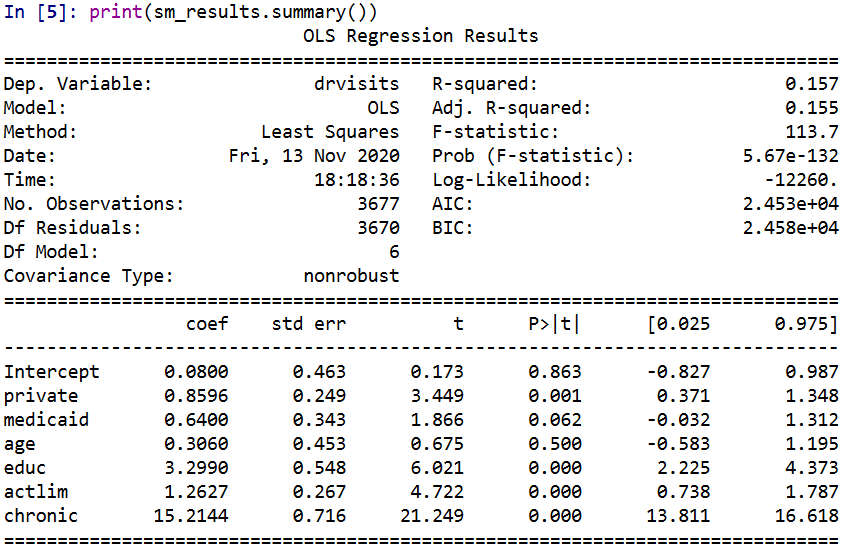Now creating the pytorch model is quite simple. For linear regression it is just one linear layer, and then setting the loss function to mean squared error. Then I create my own simple weight penalization factor in the `simp_loss` function. This takes the regression weights (not including the bias/intercept term), takes the difference between the observed weight and the rounded weight, takes the absolute value and sums those absolute values up. Then in the loop when I am fitting the model, you can see the `loss = criterion(y_pred, y_ten) + 0.4*simp_loss(model)` line. For the usual linear regression, it would just be the first criterion term. Here to add in the penalty term is super simple in pytorch, you just add it to the loss. (And you can incorporate additional penalities, the same way ala elastic-net. The Jung paper they put a penalty on the sum of coefficients as per the original lasso as well.)

Then the final part of the code after the loop is just putting the coefficients in a nicer data frame to print. And below the code snippet are the results.

``````###########################################
#Now estimating OLS model with simple coefficient
#Penalities in Pytorch

torch.manual_seed(10)

model = torch.nn.Sequential(
torch.nn.Linear(len(x_vars),1,bias=True)
)

##Initializing weights
#    model.weight = torch.nn.Parameter(coef_ten)
#    model.bias = torch.nn.Parameter(int_ten)

x_ten = torch.tensor( x_dat.to_numpy(), dtype=torch.float)
y_ten = torch.tensor( y_dat.to_numpy(), dtype=torch.float)

criterion = torch.nn.MSELoss(reduction='mean')

def simp_loss(mod):
dif = mod.weight - torch.round(mod.weight)
return dif.abs().sum()

for t in range(100000):
#Forward pass
y_pred = model(x_ten)
#Loss
loss = criterion(y_pred, y_ten) + 0.4*simp_loss(model)
if t % 1000 == 99:
print(f'iter: {t}, loss: {loss.item()}')
loss.backward()
optimizer.step()

#Making a nice dataframe of coefficients

coef_vars = ['Inter'] + x_vars
vals = list(model.bias.detach().numpy()) + list(model.weight.detach().numpy()[0,:])
res = pd.DataFrame(zip(coef_vars, vals), columns=['Var','Coef'])
print( res )
###########################################``````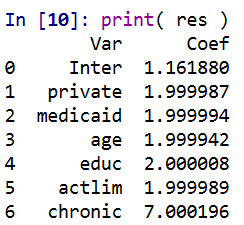Here I did not round the coefficients, so you can see that they are not exactly integer values, but are very close. So this will result in a lower loss than taking the usual linear regression coefficients and rounding them like in the noted Jung paper. It is a more direct approach. Also note that the intercept is not close to an integer value. I did not include the intercept in my penalty term. You could if you wanted to, but for most examples I don’t think it makes much sense to do that.

But one of the things that I have noticed playing around with pytorch more is that it is very difficult to get random initialized weights to converge to the same solution. That identification problem I mentioned earlier. One way is instead of using random initialized weights, is to initialize them to some reasonable values. If you uncomment the lines `with torch.no_grad():` in the above code and initialize the weights to start from the unregularized OLS solution, it converges much faster, has a slightly smaller mean square error term, and results in these effects: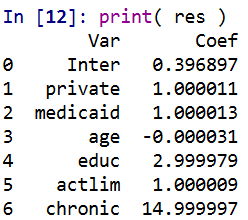So you can see in that solution it is exactly the same as rounding the initial OLS solution (ignoring the intercept again). But that may not always be the case. For example if actlim (activity limitations) and educ (education) had a very high correlation, it may be rounding both down is too big a hit to the fit of the equation, so one may go down and one go up. (You need to estimate the equation to know if things like that will occur.)

And that is all folks! While if I were sharing this more broadly, I would likely make a statsmodel like interface (and it appears they use cvxopt under the hood) instead of pytorch, it is very simple to amend pytorch to return simple weights, just add in the penalty to the loss function. Works the same way for lasso/ridge as it does for the simple weights example I give here.

Next up I want to try to figure out autograd in pytorch good enough to give standard errors for these various regression models I am estimating. While I don’t think hypothesis testing makes sense for these various models I am sharing, seeing a standard error that is very high may have prognostic value. In this case, if you had a very high standard error relative to the simple coefficient, it might suggest you should rescale the variable a different way or drop it entirely.

Also for this example, to be simple in the field it would not only need simple coefficients, but simple inputs as well. Wondering if there is a way to add in threshold layers in deep learning to automatically figure out the best way to make the inputs binary (e.g. above 70, educ below 10, etc.) instead of doing min/max scaling of the inputs.